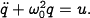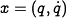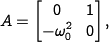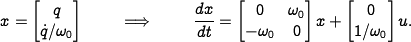# Difference between revisions of "FAQ: In Example 5.3, why is the A matrix in the given form?"

(Contributed by R. M. Murray, 5 Apr 08)

In Example 5.3, we consider the state space representation of the modelIf we choose the state vector to bethen the dynamics matrix for the system is given bywhich is different than the form listed. Instead, we can use a normalized set of coordinatesA similiar form can also be obtained by rescaling time by, as described in Example 2.6.